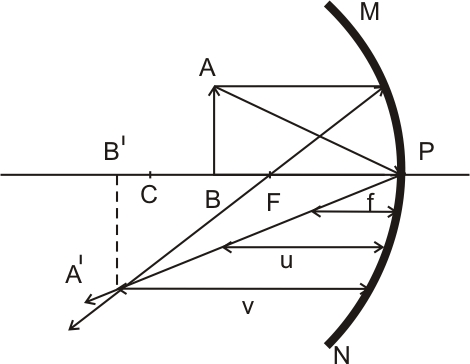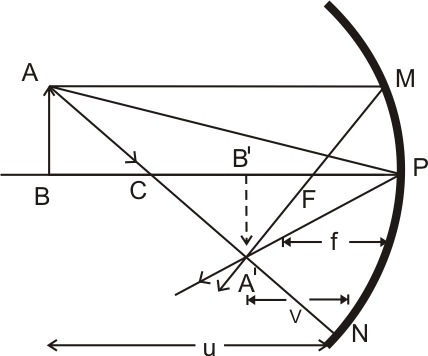# (a) Draw a ray diagram to show image formation when the concave mirror produces a real, inverted and magnified image of the object. (b) Obtain the mirror formula and write the expression for the linear magnification. (c) Explain two advantages of a reflecting telescope over a refracting telescope.

a)a concave mirror produces a real, inverted and magnified image for the object placed between F and C
b)Triangle A 'B' F and MPF are similar
Therefore,

or
Since the right-angled triangle and ABP are also similar, Therefore,

From equation (1) and (2)

Apply the sign convention
That is

using the relation equation (3) become

This relation is known as mirror equation
where, v = image distance
u = object distances
f = focal length
Linear magnification
it is the ratio of the size of the image to the size of the object it is denoted by m.

where
highest of the image
h = highest of the object
c)i) reflecting telescope is free from the chromatic aberration.
ii) In reflecting telescope spherical aberration is reduced by using the parabolic reflecting surface.
iii) Mechanical support is easier compared to the refracting telescope.

## Related Chapters

### Preparation Products

##### Knockout NEET July 2020

An exhaustive E-learning program for the complete preparation of NEET..

₹ 15999/- ₹ 6999/-
##### Rank Booster NEET 2020

This course will help student to be better prepared and study in the right direction for NEET..

₹ 9999/- ₹ 4999/-
##### Knockout JEE Main July 2020

An exhaustive E-learning program for the complete preparation of JEE Main..

₹ 12999/- ₹ 6999/-
##### Test Series NEET July 2020

Take chapter-wise, subject-wise and Complete syllabus mock tests and get in depth analysis of your test..

₹ 4999/- ₹ 2999/-